# T. Hutchcroft & M. Tointon: Non-triviality of the phase transition for percolation on finite transitive graphs

Abstract: We prove that if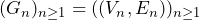is a sequence of finite, vertex-transitive graphs with bounded degrees and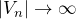that is at least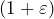-dimensional for some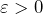in the sense that the diameter of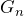is smaller than the volume ofby a power, then this sequence of graphs has a non-trivial phase transition for Bernoulli bond percolation, in the sense that giant clusters exist with high probability when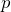is sufficiently large. Our proofs rely crucially on the quantitative structure theory of vertex-transitive graphs as developed in recent work of Tessera and Tointon, which very roughly says that for each transitive graph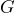and dimension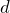there is a scale such that“looks at least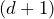-dimensional” below that scale and “looks like a nilpotent group of dimension at most” above that scale.

In the first half of the talk, Tom will overview the problem, give a high-level overview of the proof, and describe how the recent work of Duminil-Copin, Goswami, Raoufi, Severo, and Yadin can be adapted to yield the non-triviality of the phase transition for finite graphs satisfying a 12-dimensional isoperimetric condition. In the second half, Matt will give a brief survey of the quantitative structure theory of transitive graphs, describe how we prove the theorem for Cayley graphs of Abelian and nilpotent groups, and finally how we patch together the high-dimensional and nilpotent analyses at the relevant crossover scale if necessary.

This talk is based on the preprint https://arxiv.org/abs/2104.05607.

## Video